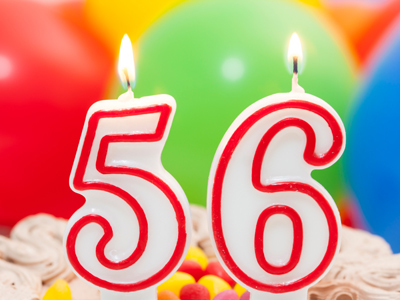8 × 7 = 56.

# 8 Times Table

If you are working your way through these 11-Plus times tables quizzes then you'll have noticed that the larger the numbers, the harder the times tables become. Learning the 8 times table until you know the answer before you even think about it will really help you. It'll come in handy in many situations, from maths lessons at school to shopping trips in everyday life.

The eight times table means multiplying numbers by 8. There are several ways to work this out. You could multiply the number by 10 and then subtract double the number. Or you could double the number three times. But the quickest way is to learn your times tables by heart.

Once you know your eight times table, keep coming back to this quiz to test yourself. The more often you practise, the better you will get. Take your time and choose your answers carefully. Good luck!

1.
8 × 6 = ?
48
58
38
78
To multiply 8 x 6, first times it by 5 and then add 8
2.
8 × 9 = ?
72
62
52
42
To multiply 8 x 9, you can times it by 10 and then subtract 8
3.
8 × 5 = ?
30
50
60
40
To multiply any number by 5, simply times it by 10 and then halve your answer
4.
8 × 12 = ?
96
90
88
92
8 x 12 is 8 x 10 (80) plus 8 x 2 (16). 80 + 16 = 96
5.
8 × 2 = ?
26
36
16
12
In a game of chess each player starts with 16 pieces
6.
8 × 11 = ?
86
88
92
99
Most pianos have 88 keys - 52 white and 36 black
7.
8 × 7 = ?
66
56
46
86
One way you could work this one out is to multiply 7 x 10 = 70 and then take away 7 x 2 = 14. 70 - 14 = 56
8.
8 × 3 = ?
44
34
14
24
8 x 3 is the same as 4 x 6 or 2 x 12
9.
8 × 8 = ?
54
44
64
34
A chessboard and a draughtsboard both have 64 squares
10.
8 × 4 = ?
22
32
42
52
Adults have 32 teeth - assuming they haven't lost any!
Author:  Frank Evans